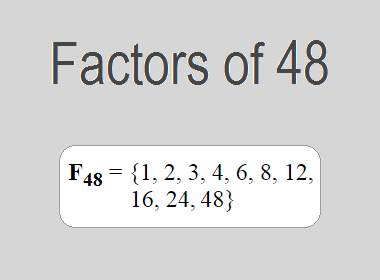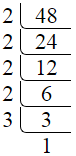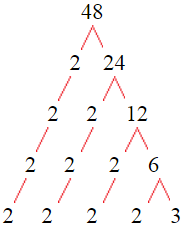# Factors of 48The factors of 48 are 1, 2, 3, 4, 6, 8, 12, 16, 24, and 48 i.e. F48 = {1, 2, 3, 4, 6, 8, 12, 16, 24, 48}. The factors of 48 are all the numbers that can divide 48 without leaving a remainder.

We can check if these numbers are factors of 48 by dividing 48 by each of them. If the result is a whole number, then the number is a factor of 48. Let's do this for each of the numbers listed above:

·        1 is a factor of 48 because 48 divided by 1 is 48.

·        2 is a factor of 48 because 48 divided by 2 is 24.

·        3 is a factor of 48 because 48 divided by 3 is 16.

·        4 is a factor of 48 because 48 divided by 4 is 12.

·        6 is a factor of 48 because 48 divided by 6 is 8.

·        8 is a factor of 48 because 48 divided by 8 is 6.

·        12 is a factor of 48 because 48 divided by 12 is 4.

·        16 is a factor of 48 because 48 divided by 16 is 3.

·        24 is a factor of 48 because 48 divided by 24 is 2.

·        48 is a factor of 48 because 48 divided by 48 is 1.

## How to Find Factors of 48?

1 and the number itself are the factors of every number. So, 1 and 48 are two factors of 48. To find the other factors of 48, we can start by dividing 48 by the numbers between 1 and 48. If we divide 48 by 2, we get a remainder of 0. Therefore, 2 is a factor of 48. If we divide 48 by 3, we get a remainder of 0. Therefore, 3 is also a factor of 48.

Next, we can check if 4 is a factor of 48. If we divide 48 by 4, we get a remainder of 0. Therefore, 4 is also a factor of 48. If we divide 48 by 5, we get a remainder of 3. Therefore, 5 is not a factor of 48. We can continue this process for all the possible factors of 48.

Through this process, we can find that the factors of 48 are 1, 2, 3, 4, 6, 8, 12, 16, 24, and 48. These are the only numbers that can divide 48 without leaving a remainder.

********************

********************

## Properties of the Factors of 48

The factors of 48 have some interesting properties. One of the properties is that the sum of the factors of 48 is equal to 124. We can see this by adding all the factors of 48 together:

1 + 2 + 3 + 4 + 6 + 8 + 12 + 16 + 24 + 48 = 124

Another property of the factors of 48 is that the prime factors of 48 are 2 and 3 only.

## Applications of the Factors of 48

The factors of 48 have several applications in mathematics. One of the applications is in finding the highest common factor (HCF) of two or more numbers. The HCF is the largest factor that two or more numbers have in common. For example, to find the HCF of 48 and 40, we need to find the factors of both numbers and identify the largest factor they have in common. The factors of 48 are 1, 2, 3, 4, 6, 8, 12, 16, 24, and 48. The factors of 40 are 1, 2, 4, 5, 8, 10, 20, and 40. The largest factor that they have in common is 8. Therefore, the HCF of 48 and 40 is 8.

Another application of the factors of 48 is in prime factorization. Prime factorization is the process of expressing a number as the product of its prime factors. The prime factors of 48 are 2 and 3. We can express 48 as:

48 = 2 × 2 × 2 × 2 × 3

We can do prime factorization by division and factor tree method also. Here is the prime factorization of 48 by division method,48 = 2 × 2 × 2 × 2 × 3

Here is the prime factorization of 48 by the factor tree method,48 = 2 × 2 × 2 × 2 × 3

## Conclusion

The factors of 48 are the numbers that can divide 48 without leaving a remainder. The factors of 48 are 1, 2, 3, 4, 6, 8, 12, 16, 24, and 48. The factors of 48 have some interesting properties, such as having a sum of 124. The factors of 48 have several applications in mathematics, such as finding the highest common factor and prime factorization.